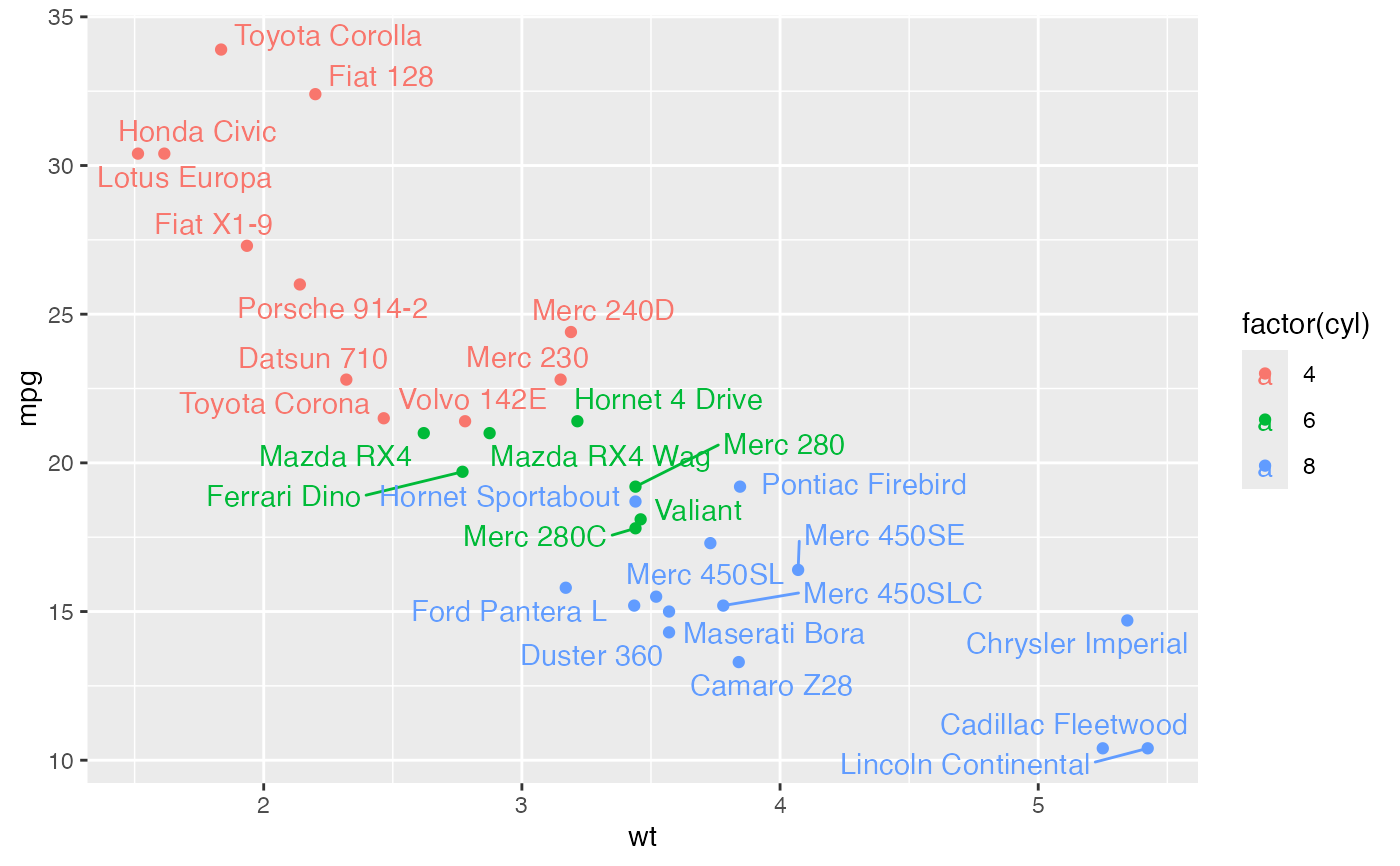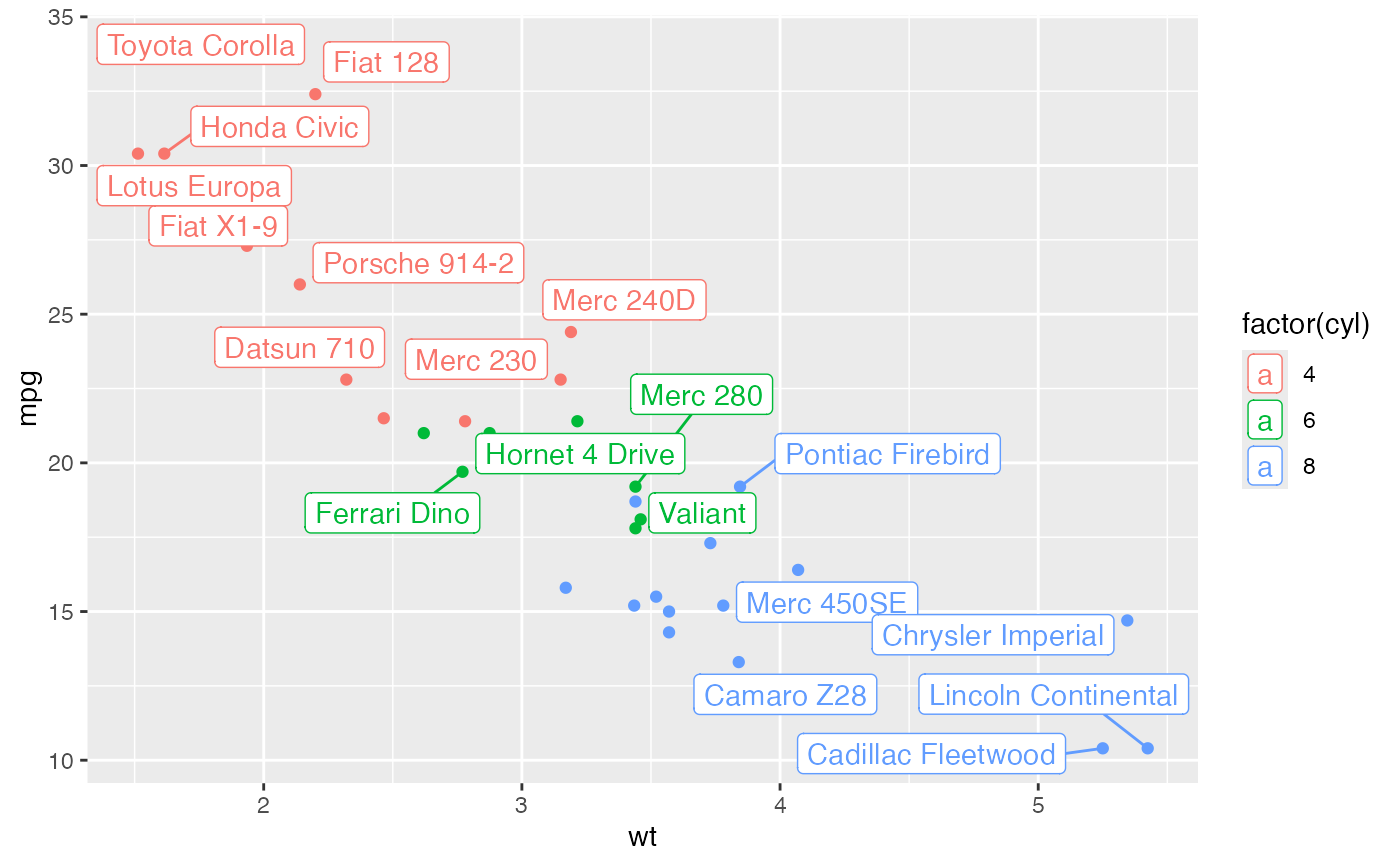geom_text_repel adds text directly to the plot. geom_label_repel draws a rectangle underneath the text, making it easier to read. The text labels repel away from each other and away from the data points.

## Usage

geom_label_repel(
mapping = NULL,
data = NULL,
stat = "identity",
position = "identity",
parse = FALSE,
...,
label.r = 0.15,
label.size = 0.25,
min.segment.length = 0.5,
arrow = NULL,
force = 1,
force_pull = 1,
max.time = 0.5,
max.iter = 10000,
max.overlaps = getOption("ggrepel.max.overlaps", default = 10),
nudge_x = 0,
nudge_y = 0,
xlim = c(NA, NA),
ylim = c(NA, NA),
na.rm = FALSE,
show.legend = NA,
direction = c("both", "y", "x"),
seed = NA,
verbose = FALSE,
inherit.aes = TRUE
)

geom_text_repel(
mapping = NULL,
data = NULL,
stat = "identity",
position = "identity",
parse = FALSE,
...,
min.segment.length = 0.5,
arrow = NULL,
force = 1,
force_pull = 1,
max.time = 0.5,
max.iter = 10000,
max.overlaps = getOption("ggrepel.max.overlaps", default = 10),
nudge_x = 0,
nudge_y = 0,
xlim = c(NA, NA),
ylim = c(NA, NA),
na.rm = FALSE,
show.legend = NA,
direction = c("both", "y", "x"),
seed = NA,
verbose = FALSE,
inherit.aes = TRUE
)

## Arguments

mapping

Set of aesthetic mappings created by aes or aes_. If specified and inherit.aes = TRUE (the default), is combined with the default mapping at the top level of the plot. You only need to supply mapping if there isn't a mapping defined for the plot.

data

A data frame. If specified, overrides the default data frame defined at the top level of the plot.

stat

The statistical transformation to use on the data for this layer, as a string.

position

Position adjustment, either as a string, or the result of a call to a position adjustment function.

parse

If TRUE, the labels will be parsed into expressions and displayed as described in ?plotmath

...

other arguments passed on to layer. There are three types of arguments you can use here:

• Aesthetics: to set an aesthetic to a fixed value, like colour = "red" or size = 3.

• Other arguments to the layer, for example you override the default stat associated with the layer.

• Other arguments passed on to the stat.

Amount of padding around bounding box, as unit or number. Defaults to 0.25. (Default unit is lines, but other units can be specified by passing unit(x, "units")).

Amount of padding around label, as unit or number. Defaults to 0.25. (Default unit is lines, but other units can be specified by passing unit(x, "units")).

Amount of padding around labeled point, as unit or number. Defaults to 0. (Default unit is lines, but other units can be specified by passing unit(x, "units")).

label.r

Radius of rounded corners, as unit or number. Defaults to 0.15. (Default unit is lines, but other units can be specified by passing unit(x, "units")).

label.size

Size of label border, in mm.

min.segment.length

Skip drawing segments shorter than this, as unit or number. Defaults to 0.5. (Default unit is lines, but other units can be specified by passing unit(x, "units")).

arrow

specification for arrow heads, as created by arrow

force

Force of repulsion between overlapping text labels. Defaults to 1.

force_pull

Force of attraction between a text label and its corresponding data point. Defaults to 1.

max.time

Maximum number of seconds to try to resolve overlaps. Defaults to 0.5.

max.iter

Maximum number of iterations to try to resolve overlaps. Defaults to 10000.

max.overlaps

Exclude text labels that overlap too many things. Defaults to 10.

nudge_x, nudge_y

Horizontal and vertical adjustments to nudge the starting position of each text label. The units for nudge_x and nudge_y are the same as for the data units on the x-axis and y-axis.

xlim, ylim

Limits for the x and y axes. Text labels will be constrained to these limits. By default, text labels are constrained to the entire plot area.

na.rm

If FALSE (the default), removes missing values with a warning. If TRUE silently removes missing values.

show.legend

logical. Should this layer be included in the legends? NA, the default, includes if any aesthetics are mapped. FALSE never includes, and TRUE always includes.

direction

"both", "x", or "y" -- direction in which to adjust position of labels

seed

Random seed passed to set.seed. Defaults to NA, which means that set.seed will not be called.

verbose

If TRUE, some diagnostics of the repel algorithm are printed

inherit.aes

If FALSE, overrides the default aesthetics, rather than combining with them. This is most useful for helper functions that define both data and aesthetics and shouldn't inherit behaviour from the default plot specification, e.g. borders.

## Details

These geoms are based on geom_text and geom_label. See the documentation for those functions for more details. Differences from those functions are noted here.

Text labels have height and width, but they are physical units, not data units. The amount of space they occupy on that plot is not constant in data units: when you resize a plot, labels stay the same size, but the size of the axes changes. The text labels are repositioned after resizing a plot.

## geom_label_repel

Currently geom_label_repel does not support the rot argument and is considerably slower than geom_text_repel. The fill aesthetic controls the background colour of the label.

## Alignment with hjust or vjust

The arguments hjust and vjust are supported, but they only control the initial positioning, so repulsive forces may disrupt alignment. Alignment with hjust will be preserved if labels only move up and down by using direction="y". For vjust, use direction="x".

## Examples


p <- ggplot(mtcars,
aes(wt, mpg, label = rownames(mtcars), colour = factor(cyl))) +
geom_point()

# Avoid overlaps by repelling text labels
p + geom_text_repel()# Labels with background
p + geom_label_repel()
#> Warning: ggrepel: 2 unlabeled data points (too many overlaps). Consider increasing max.overlapsif (FALSE) {
p + geom_text_repel(family = "Times New Roman",

p + geom_text_repel(aes(alpha=wt, size=mpg))
p + geom_label_repel(aes(fill=factor(cyl)), colour="white", segment.colour="black")

# Draw all line segments
p + geom_text_repel(min.segment.length = 0)

# Omit short line segments (default behavior)
p + geom_text_repel(min.segment.length = 0.5)

# Omit all line segments
p + geom_text_repel(segment.colour = NA)

# Repel just the labels and totally ignore the data points
p + geom_text_repel(point.size = NA)

# Hide some of the labels, but repel from all data points
mtcars$label <- rownames(mtcars) mtcars$label[1:15] <- ""
p + geom_text_repel(data = mtcars, aes(wt, mpg, label = label))

# Nudge the starting positions
p + geom_text_repel(nudge_x = ifelse(mtcars$cyl == 6, 1, 0), nudge_y = ifelse(mtcars$cyl == 6, 8, 0))

# Change the text size
p + geom_text_repel(aes(size = wt))
# Scale height of text, rather than sqrt(height)
p + geom_text_repel(aes(size = wt)) + scale_radius(range = c(3,6))

# You can display expressions by setting parse = TRUE.  The
# details of the display are described in ?plotmath, but note that
# geom_text_repel uses strings, not expressions.
p + geom_text_repel(aes(label = paste(wt, "^(", cyl, ")", sep = "")),
parse = TRUE)

p +
geom_text_repel() +
annotate(
"text", label = "plot mpg vs. wt",
x = 2, y = 15, size = 8, colour = "red"
)